or# Trigonometric Functions NCERT Solutions : Class 11 Maths

## NCERT Solutions for Class 11 Maths : Trigonometric FunctionsFilter
Filters :
Classes
•
•
•
•
•
•
•
Chapters
•
•
•
•
•
•
•
•
•
•
• 6 More
Exercises
•
•
•
•
•
•
•

Doubtnut one of the best online education platform provides free NCERT Solutions of Maths for Class 11 Trigonometric Functions which are solved by our maths experts as per the NCERT (CBSE) guidelines. We provide the solutions as video solutions in which concepts are also explained along with answer which will help students while learning , preparing for exams and in doing homework. These Solutions will help to revise complete syllabus and score more marks in examinations.

Get here free, accurate and comprehensive NCERT Solutions for Class 11 Maths Trigonometric Functions which have been reviewed by our maths counsellors as per the latest edition following up the CBSE guidelines. We provide video solutions in which solutions to all the questions of NCERT Class 11 Maths textbook are explained in step by step and detailed way.

## The Topics of the Chapter Trigonometric Functions are :

TRIGONOMETRIC EQUATIONS

GENERAL SOLUTION OF TRIGONOMETRIC EQUATIONS

FORMULAE TO TRANSFORM THE SUM OR DIFFERENCE INTO PRODUCT

GENERAL SOLUTION OF TRIGONOMETRIC EQUATIONS OF THE FORM

TRIGONOMETRIC RATIOS FOR SUM OR DIFFERENCE OF ANGLES

TRIGONOMETRIC RATIOS OF MULTIPLE AND SUB-MULTIPLE ANGLES

TRIGONOMETRIC RATIOS OF A IN TERMS OF THAT OF ANGLE A/2

TRIGONOMETRIC RATIOS OF SUM & DIFFERENCE OF TWO ANGLES

TRIGONOMETRIC RATIOS OF ALLIED ANGLES

VARIATION IN VALUES OF TRIGONOMETRIC FUNCTIONS IN DIFFERENT QUADRANT

RELATION BETWEEN THREE SYSTEM OF MEASUREMENT OF AN ANGLE

TRIGONOMETRIC RATIOS OR FUNCTIONS

SYSTEM OF MEASUREMENT OF ANGLES

TRIGONOMETRIC EQUATIONS OF THE FORM `ACOSTHETA+BSINTHETA=C`

SIGNS OF TRIGONOMETRIC RATIOS & FUNCTIONS

INTRODUCTION

TRIGONOMETRIC RATIOS OF 2A IN TERMS OF THAT OF ANGLE A

BASIC CONCEPTS OF MENSURATION

SOME DEFINITION

THE LAW OF COSINES

PROJECTION FORMULA

THE LAWS OF `SINES OR SINE RULE`

TRIGONOMETRIC RATIOS OF ANGLE A IN TERMS OF COSA

HALF-ANGLE FORMULAS

It contains these exercises along with solved examples. All the exercises are solved in the video. Select the exercise to view the solutions exercisewise of the Chapter Trigonometric Functions:

## NCERT Solutions Class 11 Maths Chapter Trigonometric Functions Exercises:

We have covered all the exercises and also Solved examples in the videos. Along with the practise exercise Students should also practise solved examples to clear the concepts of the Trigonometric Functions. If incase you have any doubt you can watch the solutions for the given questions.Watch the solutions for the given questions in which it is also explained in the video steps to solve the questions along with answers.

In this chapter, we will learn about trigonometric functions and identities like measures of angles, the definition of trigonometric functions, applications of basic trigonometry on eliminating variables, sign and graphs of trigonometry, ratios of angles, etc. We will be discussing various applications in different questions. Trigonometry is not just a chapter, it’s a function so it has its application in pure trigonometry as well as in the chapters other than trigonometry, it has its application in a subject like physics. This chapter teaches about solving the complicated problem easier in just a few steps. So, learning trigonometry and using it efficiently makes the whole big process of solving the problems much easier.

NCERT textbooks provide the best and the appropriate amount of content needed by you for your academics and even for further use in competitive examinations like IIT JEE Mains and Advanced. They have their content put together by the best of teachers with years of experience and great command over the subject. NCERT provides one of the best question banks as they strictly follow the questions as per the examination pattern. The textbook provides an explanation of more than one concept in a single question so it is beneficial for you in two ways. Firstly, it provides you the opportunity to revise more than one concept through a single question and secondly, you will get to know the actual pattern of the questions which you will be dealing through in the examination. NCERT textbook has covered each and every topic and explained it well for your understanding. The NCERT class 11 book provides explanations utilizing coloured pictures, graphs and tables for a more vivid understanding of the topic and also it does not make the content look boring ‘black’ themed. NCERT book provides questions after every topic and some question in between the text to keep you curious and eager to know more about the chapter and topics. It provides a summary at the end of every chapter for your quick revision and several exercises for your practice.

In addition, you can visit our website Doubtnut to discover more fun and enjoyable ways of learning complex topics and tough chapters through the study material curated for students with different pace of learning. We hereby provide video lectures and tutorials that makes it even easier for you to understand the topic in a comprehensive manner. You can always post your doubtful questions over the portal and we will provide you the links in seconds, where you can find the easy solutions and appropriate explanation of the same. The solutions provided are free for everyone and we also provide the pdf format NCERT solutions, which you can access and download with just one click.

## Topics & Subtopics of NCERT Class 11 Maths Chapter 3 Trigonometric Functions

### Measurement of Angles

The word ‘trigonometry’ is derived from a Greek word. Trigonon, which means triangle and the word Metron means to measure. Hence, trigonometry means measuring the sides and angles of a triangle. The subject originally developed to solve geometric problems involving triangles.

### Angle

An angle is the measure of rotation from the direction of one ray about its initial point. The original ray called the initial side and the final position of the ray after rotation is called the terminal side of the angle. The point of rotation is called the vertex.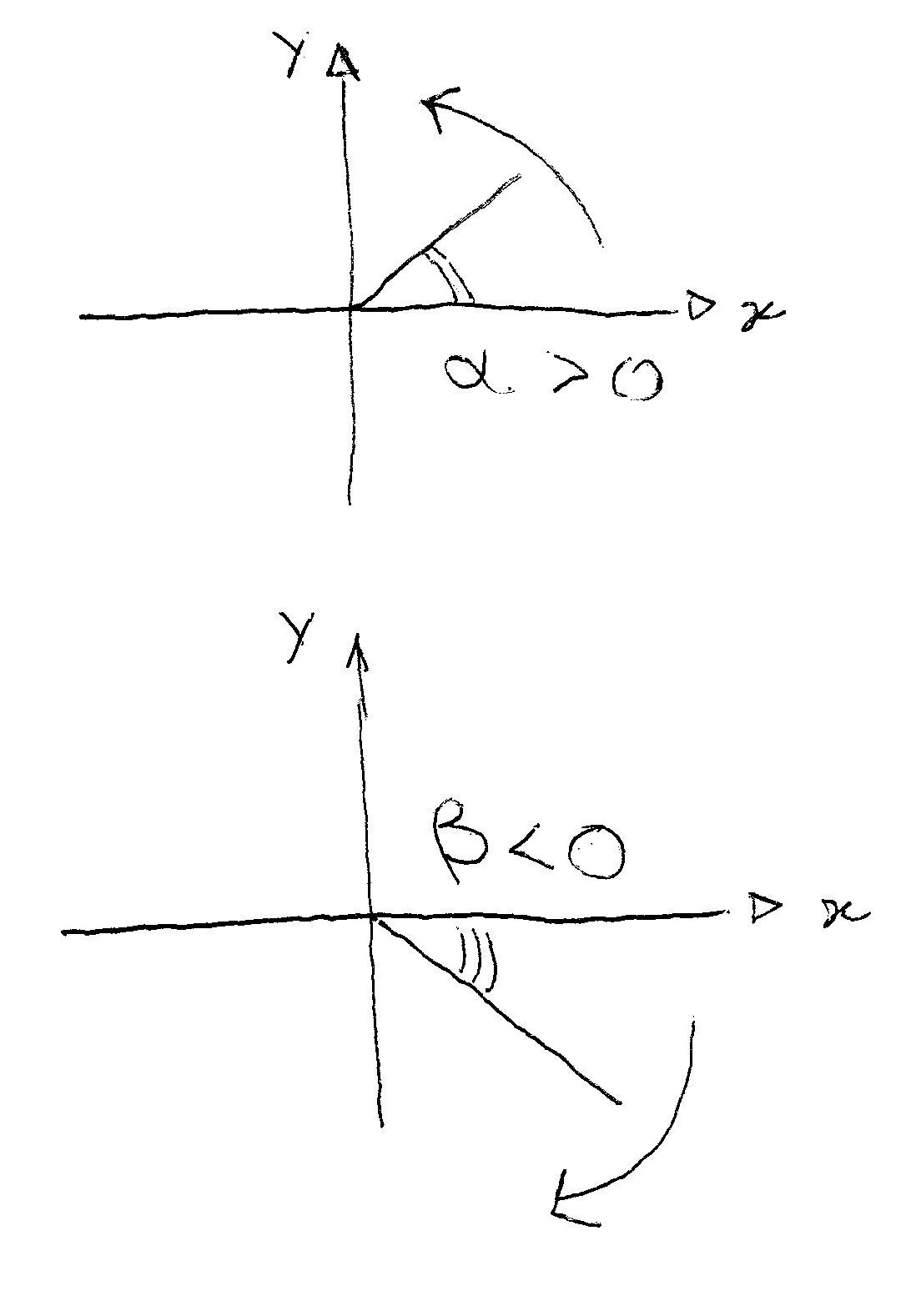NOTE: RADIAN IS A CONSTANT ANGLE

### Trigonometric Functions

An angle whose measure is greater than 0º but less than 90º is called an acute angle.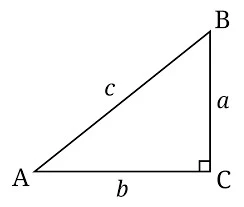BC/AB = opposite side/hypotenuse, is called sine of A and written as sinA

AC/AB = adjacent side/hypotenuse, is called the cosine of A and written as cos A.

BC/AC = opposite side / adjacent side is called the tangent of A and written as tan A

AB/BC = hypotenuse / opposite side is called cosecant of A and written as cosec A

AB/AC = hypotenuse / opposite side is called secant of A and written as sec A

AC/BC = adjacent side / opposite side is called cotangent of A and written as cot A.

### Some Values of Trigonometric Ratios

The values of sin, cos, tan, cot, sec and cosec of angles 0º, 30º, 45º, 60º, 90º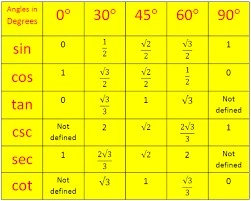### Trigonometric Identities

Trigonometric identities are equalities that involve trigonometric functions that are true for every single value of the occurring variables. In other words, they are equations that hold true regardless of the value of the angles being chosen.

Trigonometric identities are as follows:

1. sin2 A + cos2A =1 this implies that cos2 A = 1- sin2A

or sin2A= 1-cos 2A

1. 1+ tan2 A = sec 2 A this implies that sec2A-tan2 A=1
2. cot 2 A +1 = cosec 2A this implies that

cosec2 A – cot2 A = 1

1. tan A = sinA cosA and cotA = cosA / sinA
2. Fundamental inequalities:

for 0

0 < cosA < sinA/A <1/cos A

1. IT IS POSSIBLE TO EXPRESS TRIGONOMETRICAL RATIOS IN TERMS OF ANY ONE OF THEM AS:

sinÏ´= 1/1+cot2 Ï´

cosÏ´= cotÏ´/ 1+cot2 Ï´ , tan Ï´= 1/ cotÏ´

cosec Ï´= 1+ cot2 Ï´ , secÏ´ = 1+cot2Ï´/ cotÏ´

That is all trigonometric functions have been expressed in terms of cot Ï´

Similarly, we can express all trigonometric functions in other trigonometric ratios.

## Application of Basic Trigonometry on Eliminating Variables or Parameters and Geometry

Parameters are those values, which could vary example Ï´ if parameter could take any value as:

Ï´ = 0° 30° 45° 90° 60° 120°,....

Thus to eliminate this we need to use basic trigonometric functions and formulae,

Example:

If asec É‘ – ctan É‘=d and bsec É‘ +dtan É‘=c, then eliminate É‘ from above equations.

Solution:

Here,  asec É‘ – ctan É‘=d and bsec É‘ +dtan É‘=c could be written as

a= dcos É‘ + csin É‘                ...1

b= ccosÉ‘ – dsinÉ‘                   ....2

On squaring and addning equations 1 and 2, we get

a2 + b2 = (d cosÉ‘ + c sinÉ‘)2 + (c cos É‘ – dsinÉ‘)2

This implies a2+b2 = d2 cos2 É‘+ c2 sin2 É‘ +2dc cosÉ‘sinÉ‘ +c2 cos2 É‘ + d2 sin2É‘ -2dc cos É‘sinÉ‘

= d2((cos2É‘ + sin2 É‘) + c2(sin2 É‘ + cos2 É‘)

Therefore a2+b2=c2+d2

### Signs and Graph of Trigonometric Functions

#### Signs of Trigonometric Functions

The signs of the trigonometric ratios of an angle depending on the quadrant in which the terminal side of the angle lies.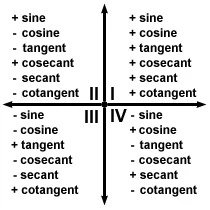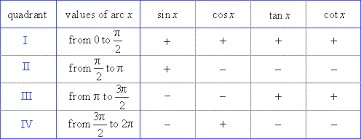Variation in The Values of Trigonometric Functions in Different Quadrants

We observe that in the first quadrant, as x increase from 0 to π/2, sin x increases from 0 to 1 and in the second quadrant as x increases from π/2 to π, sin x decreases from 1 to 0.

In the third quadrant, as x increases from π to 3π/2, sin x decreases from 0 to -1 and finally, in the fourth quadrant sin x increases from -1 to 0 as x increases from 3π/2 to 2π.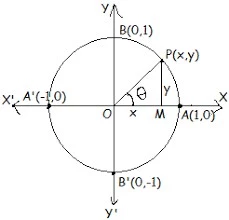### Graphs of Trigonometric Functions

As in the case of algebraic functions, we can have some idea about the nature of a trigonometric function by its graphs.

Graphs have many important applications in mathematical problems. We shall discuss the graphs of trigonometrical functions. We know that sinx , cos x, secx, and cosecx are periodic functions with period 2π and tanx and cot x are trigonometric functions with period π . Also, if the period of function f(x) is T, then period of f(ax+b) is T /Ë©aË©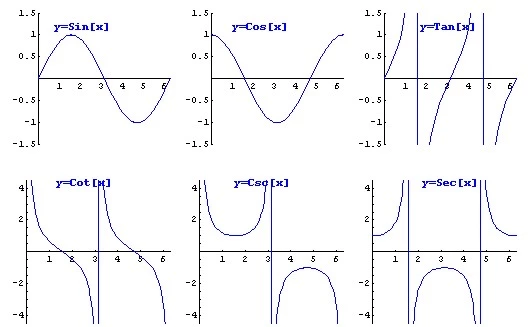### Properties of Tangent Function

y = a tan[b(x+h)]+k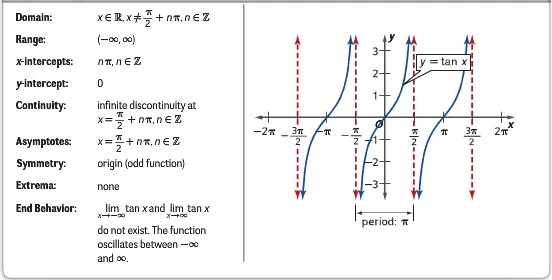### Properties of cotangent function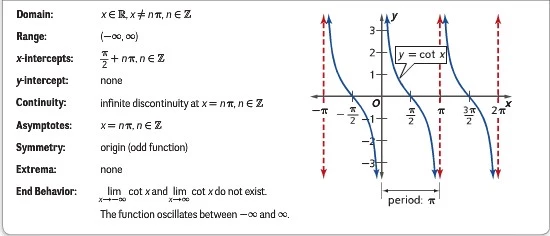### Trigonometric Ratios of an Angle

Considering the system of rectangular coordinates axis dividing the plane into four quadrants. The positive values of the trigonometric ratios in the various quadrants are shown; the signs of other ratios may be derived.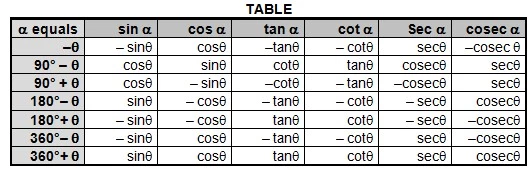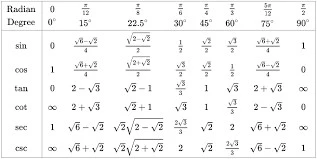Benefits of Doubtnut

1.  Doubtnut provide solutions to the NCERT questions and explanations of the topics mentioned in the chapters.
2. Doubtnut presents video lectures for the easy explanation and better understanding of the concepts, theories, methods, and formulas. You will get to learn the concepts in a subtle way as it is very well-known that videos provide a better understanding than just reading.
3. We also provide links of the solutions for the questions posted by you on our website and we take the most demanded topic for the next video by your comments and will always welcome any change in the pattern of teaching or the content as needed by you. You can post your comment about any kind of queries and doubts and we will surely acknowledge it.
4. Doubtnut provides the learning material in the easiest language possible for everyone to understand and get hold of it. We do not charge any registration fee for the solutions provided. The solutions can be accessed for free by anyone from anywhere in the world.
5. We trust in providing the immersive learning experience to our readers in the best way possible to us. For any changes, you can always reach to us and we will take care of it.